# Electrochemical Impedance Spectroscopy of Tap Water

January 6, 2020 by Meng Li

## Introduction

Electrochemical impedance spectroscopy (EIS) is a popular non-destructive technique to characterize the physicochemical properties of materials, particularly at the solid-liquid (or electrode-electrolyte) interface. Despite the complexity of the measured data, EIS can be used to study many electrochemical devices (batteries, supercapacitors and fuel cells), dielectric coatings, as well as microfluidic chips. The purpose of this blog post is to demonstrate how to perform EIS with the MFIA Impedance Analyzer (or the MFLI Lock-in Amplifier with the MF-IA option), using an easily obtainable sample of tap water.

## Background and Setup

In the liquid state, the measurement setup is quite different from the solid state. This naturally originates from the challenge in confining the geometry of DUT. In this post, we use a capacitive sensing probe for demonstration; inductive sensors are not covered here . As the name implies, the sensing part of the probe is a pair of parallel (coated) metal plates, which is carefully designed to have a cell constant (k) of 1 cm . The conductivity (σ), which is usually linearly proportional to the ionic concentration in dilute strong electrolytes, can then be easily calculated from the conductance (G).

$G=\sigma\frac{A}{d}=\sigma k$

Due to the nature of the capacitor that blocks DC current, the measurement is carried out via AC test signal, generated from the HCur BNC port on the MFIA. Here we connect a single-ended conductivity probe using the setup as shown in Figure 1. 4-terminal measurement is conducted by pinning the HCur, LCur, HPot and LPot ports together at the BNC end of the probe. Before starting the measurement, the sensing part of the probe was soaked into tap water overnight to ensure complete wetting.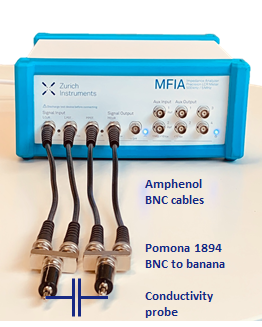Figure 1: A Scheme showing the setup of the MFIA connected to a single-ended conductivity probe.

## Electrochemical Impedance Spectroscopy

We begin the experiment by setting the AC test voltage as 100 mV in amplitude and sweep the frequency from 10 mHz to 5 MHz using the "Sweeper" tool in LabOne software. As shown in figure 2, the measured amplitude and phase (Bode plot) can be conveniently displayed at the same time by simply checking the "Dual Plot" button. To toggle into Nyquist plot (figure 3), one can simply select "On-Invert" in the drop-down menu next to "XY Mode", choose "Impedance 1 Real (Z)" as the "X Signal", and "IA Imag (Z)" as Y in the "Vertical Axis Groups".

In Figure 2, the amplitude decreases at low frequency with a slope slightly higher than -1, becomes flatten within 100 Hz to 10 kHz range and returns to a -1 slope again at high frequency. It is worth noticing that the amplitude decreases by 3 orders of magnitude during the sweep. Thanks to the auto-ranging function of the MFIA, the sweep can be done seamlessly without manually adjusting the input ranges.

In terms of phase, we see the 100 Hz to 10 kHz region corresponds to a small, but non-zero angle (maximum -1.2 deg at 1.4 kHz). In addition, the trace never reaches -90 deg (purely capacitive) in the measured frequency range. The result clearly distinguishes from a simple RC circuit model, that is frequently used in solid-state DUTs. This is very interesting and worth looking at in depth.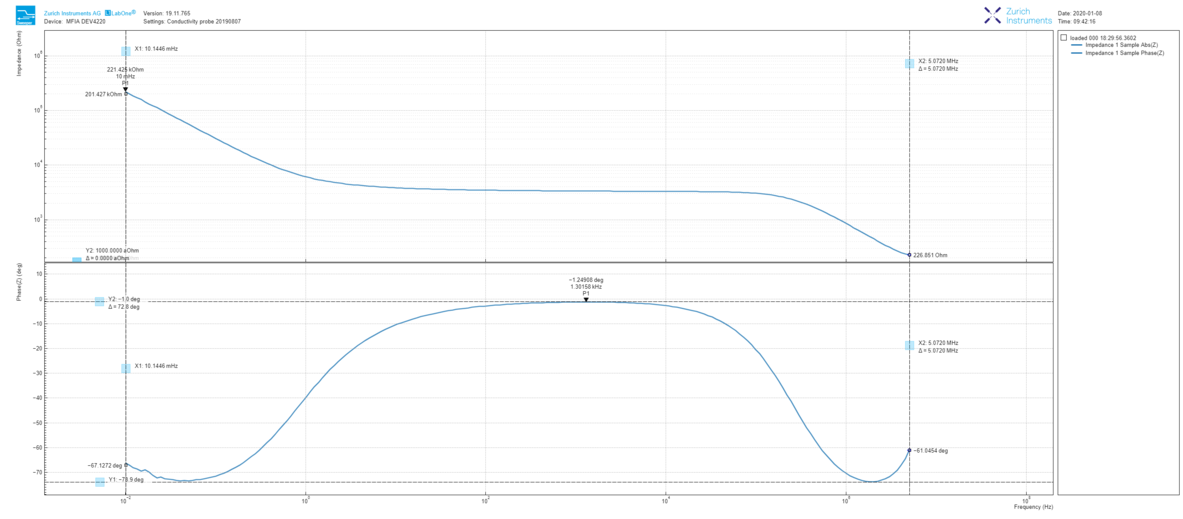Figure 2: Dual Bode plot derived from the LabOne Sweeper tool, showing both the amplitude and phase of the measured tap water impedance from 10 mHz to 5 MHz.

To obtain more statistics, we set continuous sweeps over a weekend. Note that starting from the LabOne 19.05 release, measurements from the Sweeper (as well as DAQ and Scope) module can be saved in .h5 format, allowing convenient reloading for comparison at a later stage. By checking the Auto save button, each sweep can be stored in either a single or multiple file(s) automatically. As shown by the Nyquist plot in Figure 3, 15 measurements are taken and reloaded into LabOne in sequential order. Over time, the impedance of tap water gradually decreases (real-part shifting to the left) due mostly to slow water evaporation as well as CO2 dissolution from the room atmosphere .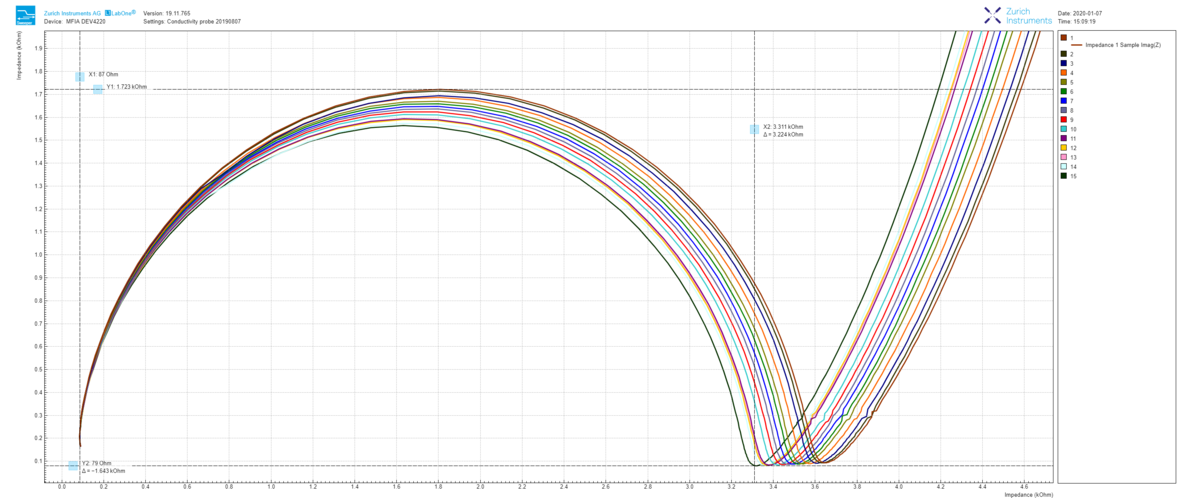Figure 3: 15 continuous impedance measurements reloaded into LabOne Sweeper module, and zoomed-in at the high-frequency end in the Nyquist plot.

## Equivalent Circuit Modeling

To get a meaningful explanation, we proceed by fitting the impedance spectrum with the inset circuit model in Figure 4. The MFIA can export sweeper data in .csv, .m (MATLAB), .h5 (HDF5) and .txt formats, which can be loaded into circuit modeling programs. It should be mentioned that, before the modeling, one should also perform a Kramers-Kronig analysis to check the linearity, casualty, and stability of the measured data . Fitting results are listed in Table 1.

Name Value Error
R1  80.45 Ohm 3.2%
R2  3320 Ohm 0.29%
C  1.834×10-10 F 0.58%
Q  4.59×10-5 Ohm-1 sn 0.97%
n 0.845 0.51%
Zw 1.184×106 Ohm s-0.5 11%
τ 7.40 s 9.6%
α 0.780 5.6%

Table 1: Fitting results of the impedance spectrum in figure 4. Q and n are from the CPE; Zw, τ, and α are from the Warburg short element. For a detailed explanation of the parameters, readers are kindly referred to .

We use a modified Randles circuit, where R1 represents the resistance in the solid phase, coming mainly from the parasitics of the connecting cables and the internal resistance of the probe. The influence from the first term can be minimized by performing user compensation with electrolyte in a known conductivity for calibration. And the latter can be done via connecting the 4 terminals as close as possible to the capacitor plates (DUT), rather than at the BNC breakout adapter. In the middle of the circuit is the electrochemical double layer formed at the solid-liquid interface. Note that, a non-ideal Warburg short (Ws) term is used together with a constant-phase element (CPE), replacing typical double-layer capacitance commonly seen elsewhere . Such a complex behavior is likely due to the fact that tap water contains multiple types of ions and even non-ionic species [5, 6]. Since the CPE does not reveal a perfect "-90 deg", the slope in Bode plot slightly deviates from -1 at low frequency. On the right side of the model (see inset of Figure 4), the R2||C is the resistance and capacitance of tap water in the liquid phase. This capacitance correlates nicely to the -1 slope at high frequency.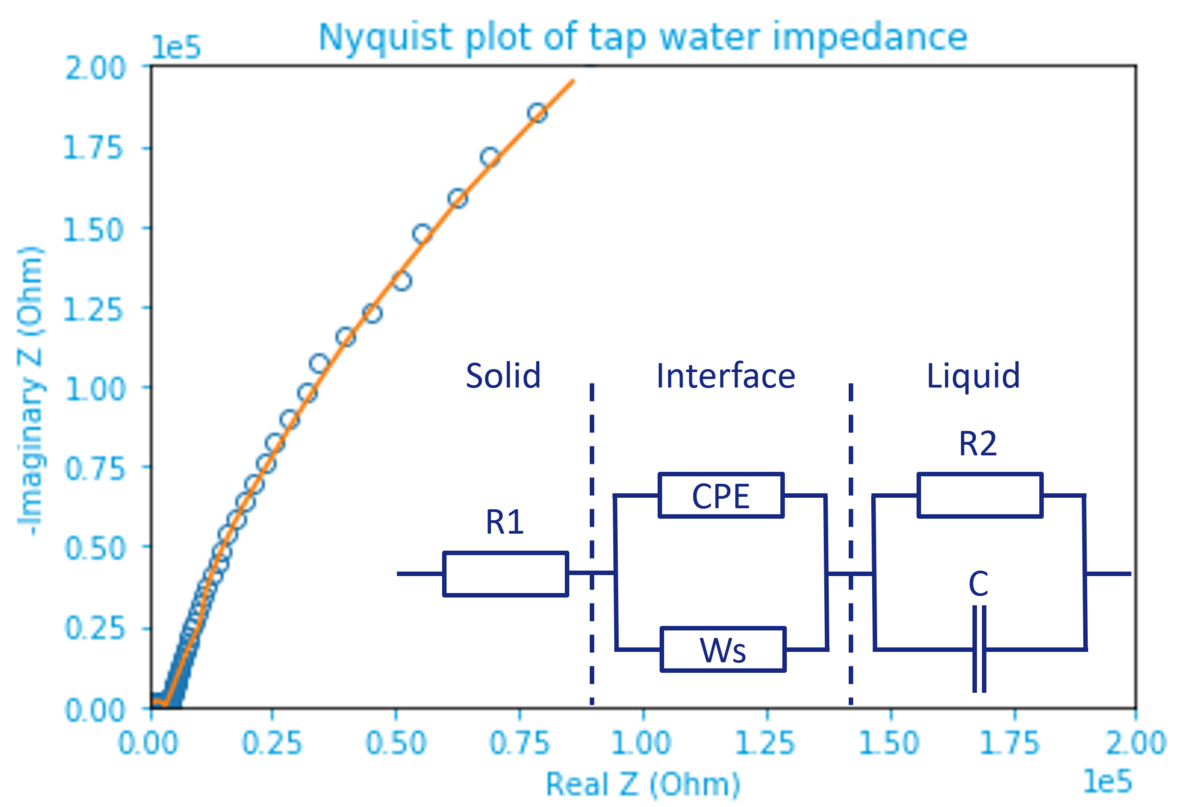Figure 4: Nyquist plot of tap water impedance fitted by the inset equivalent circuit model. Raw data are marked by open dots and the fitting result represented by an orange line. The inset circuit shows solid(electrode)-liquid(electrolyte) (inter)phases separated by dashed lines.

Since this blog post aims at studying the conductance of liquid (tap water), we focus here on the middle frequency (100 - 10 kHz) region, where the resistance of the liquid phase (R2) dominates. An easy way is to understand the complicated circuit is to imagine that the impact from Ws and C is small, such that it can be simplified as R1+R2+CPE. The CPE creates only a small shift in the phase, making it slightly "capacitive" (below zero) at around 1.4 kHz. Therefore, the conductivity probe behaves similar to a resistor. The measured impedance (at the lowest phase) of 3347 Ohm in Bode plot matches very well with the fitted solution resistance R2, i.e., 3320 Ohm. Using the equation above, we can find the conductivity of tap water as 30 mS/m, agreeing with the reported range .

In fact, some conductivity probe manufacturers specify a fixed frequency (e.g. 1 kHz) for simplicity. Since the impedance spectrum of tap water used here shows a broad flat region between 100 and 10 kHz, this can be a good approximation. However, when the electrode-electrolyte interface (due to pronounced double-layer effect as in supercapacitors) or electrolyte itself (due to capacitive solutes such as cells) becomes more capacitive, such a simplification becomes invalid. Instead, one should carefully extract the electrolyte resistance by performing circuit modeling from the measured impedance spectrum. This clearly shows the advantage of sweeping over measurement at a fixed frequency.

## Real-Time Impedance Monitoring

Finally, let's now have a look at the real-time impedance evolution. The MFIA can record and stream the measured data using the Plotter tool in LabOne or one of the five included APIs (C, LabVIEW, MATLAB, Python and .NET). The data transfer rate of the instrument can be set up to 107 KSa/s, which is much faster than Sa/s range as in typical conductivity probes. This allows the observation of fast-changing processes in the liquid. As an example (Figure 5), after adding a few NaCl salt grains into the measured tap water, the conductance increases to 970 μS (97 mS/m in conductivity, which exceeds drinking water range , and the phase drops to -4.7 deg within 8 seconds. This supports the claim in the previous section that impedance measurement at a fixed frequency is less than ideal.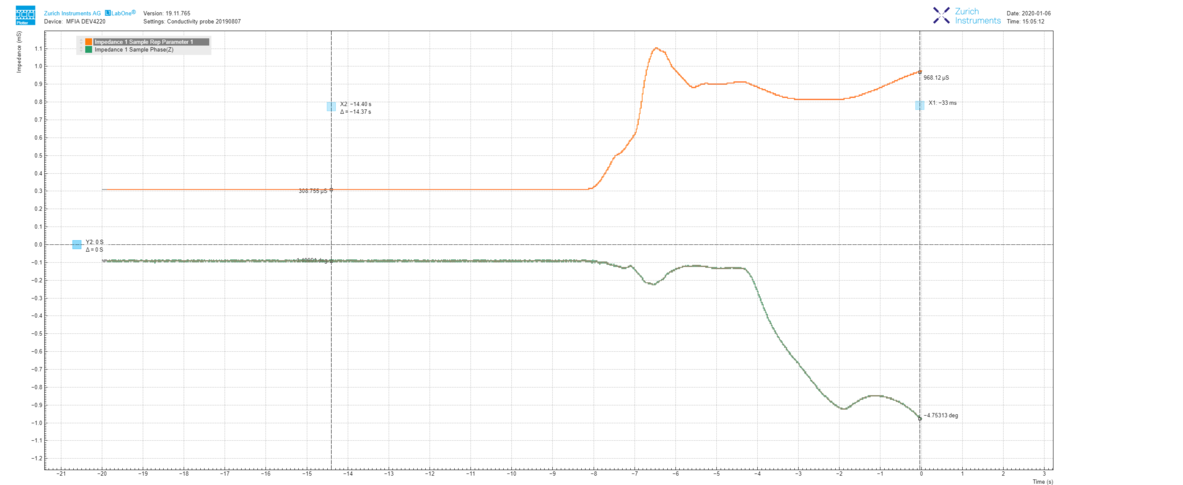Figure 5: LabOne Plotter data showing the evolution of impedance measured at 1 kHz. The increase in conductance, as well as the decrease in phase, is caused by the addition of NaCl salt.

## Conclusion

This blog post describes how to set up the MFIA for measuring EIS in liquid over a wide frequency range, rather than at a sub-optimized fixed frequency. Once the frequency of interest is determined, the MFIA can help to monitor fast processes in the time domain. This suits to study the electrolyte performance for supercapacitor, battery, and microfluidic applications. Furthermore, thanks to its fast response and good accuracy, the MFIA is also an ideal candidate for impedance-based sensor applications. Note that to get more reliable measurements over a long time (e.g., extending the measurement frequency to 1 mHz), additional temperature and atmosphere control would be recommended. For further information on EIS measurements, please get in touch with us.

## References

 Bhat, S. (2005). Salinity (conductivity) sensor based on parallel plate capacitors. Graduate Theses and Dissertations. http://scholarcommons.usf.edu/etd/2784

 Li, M et al. (2018). Pseudocapacitive coating for effective capacitive deionization. ACS Applied Materials & Interfaces, 10(3), 2442-2450.

 Bisquert, J et al. (2000). Doubling exponent models for the analysis of porous film electrodes by impedance. Relaxation of TiO2 nanoporous in aqueous solution. The Journal of Physical Chemistry B, 104(10), 2287-2298.

 Sammer, M et al. (2016). Strong Gradients in Weak Magnetic Fields Induce DOLLOP Formation in Tap Water. Water, 8(3), 79.

 Sammer, M et al. (2019). Biomass measurement of living Lumbriculus variegatus with impedance spectroscopy. Journal of Electrical Bioimpedance, 5(1), 92-98.